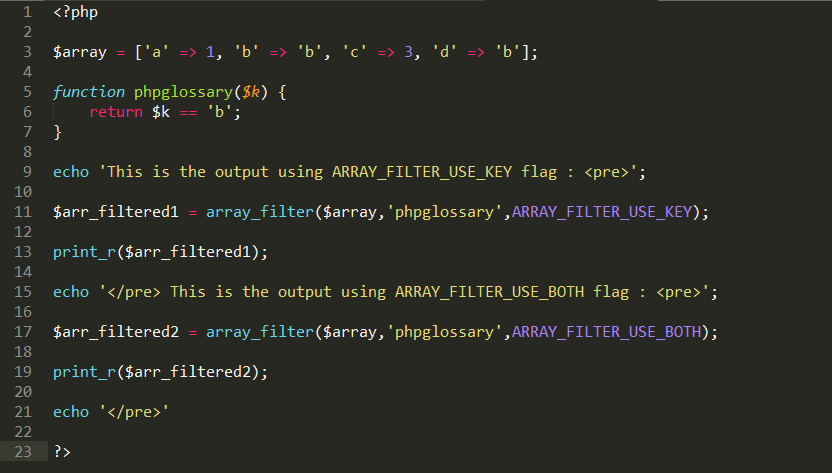functions

# How to use array_filter() function in php ?The array_filter() function use to filters the elements of an array using a callback function. This function accepts two parameters and one flag. The first parameter is required which is to be an array

The array_filter() function use to filters the elements of an array using a callback function.

This function accepts two parameters and one flag. The first parameter is required which is to be an array, the second parameter is optional which is to be a callback function if passed and the last flag is also optional. The flags names are ARRAY_FILTER_USE_KEY and ARRAY_FILTER_USE_BOTH. By default array_filter() function uses ARRAY_FILTER_USE_KEY flag to filter the array. The flags were added in PHP version 5.6.0.

The array_filter() function is used to passes each value of the array as a parameter of the callback function. If the passed callback function returns the value true, the current value from the input is returned into the result array. Array keys are preserved as they are previous.

Example :

```<?php

\$array = ['a' => 1, 'b' => 'b', 'c' => 3, 'd' => 'b'];

function phpglossary(\$k) {
return \$k == 'b';
}

echo 'This is the output using ARRAY_FILTER_USE_KEY flag : <pre>';

\$arr_filtered1 = array_filter(\$array,'phpglossary',ARRAY_FILTER_USE_KEY);

print_r(\$arr_filtered1);

echo '</pre> This is the output using ARRAY_FILTER_USE_BOTH flag : <pre>';

\$arr_filtered2 = array_filter(\$array,'phpglossary',ARRAY_FILTER_USE_BOTH);

print_r(\$arr_filtered2);

echo '</pre>'

?>```